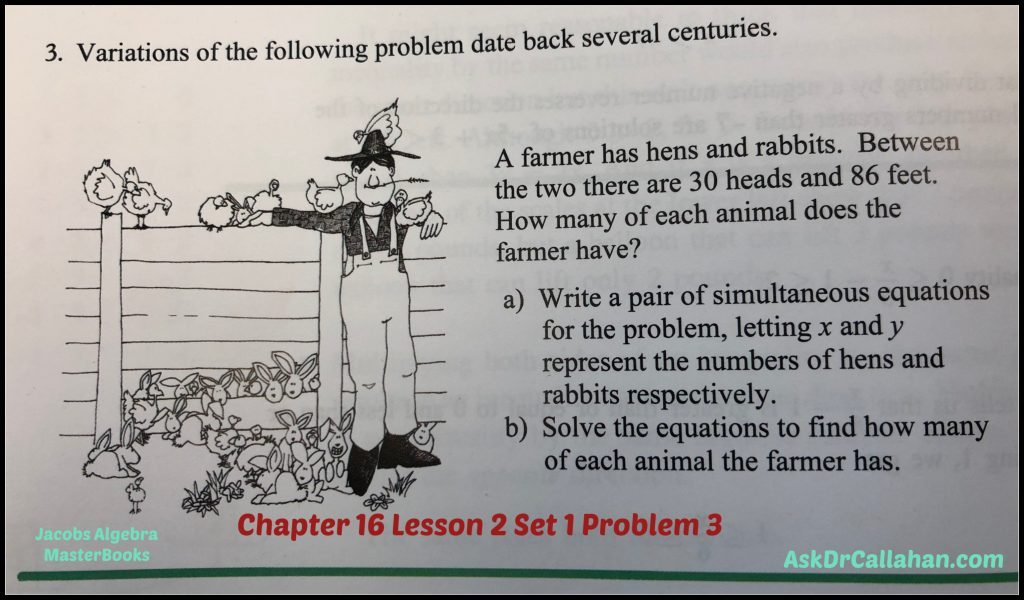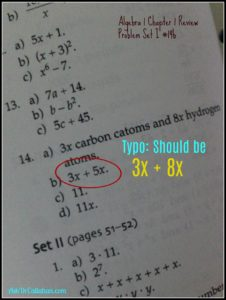Posted on

## Algebra Chapter 16 Lesson 2 Set 1 Problem #3

This is an error in the book by Master Books (ISBN: 978-0890519851) – the book with the blue cover showing the world.

The problem is discussing hens and rabbits with a total of 30 heads and 86 feet.ERROR: The solution manual is referring to the wrong problem which is related to money.

Here is how to solve. Simultaneous equations are covered in Chapter 7. So if you forget how to solve these, refer back to chapter 7.

heads = # hens + # rabbits

feet = 2 x # hens + 4x number of rabbits

So let h be the number of hens and r be the number of rabbits.

We can re-write the above as

heads = 30 = h + r

feet = 86 = 2h + 4r

30=h+r

So r = 30-h

Substitute this equation into the second equation to get

86 = 2h + 4r

86 = 2h + 4(30-h) = 2h + 120 – 4h = -2h +120

Rearrange to get

2h = 120-86 = 34

h = 17 (number of hens)

Now we can find the rabbits are

R = 30-h = 30-17 = 13

Checking our work

13 + 17 = 30 (total number of heads)

2 x 17 + 4 x 13 = 86 which are the number of feet.

Correct!

Posted on

## Algebra Ch5.1 #10x

This is an error in the Algebra Solutions Manual

In Algebra 1, chapter 5 lesson 1 problem 10x, the answer key says the answer is true for all positive integers.  But isn’t it true for all integers? 1 to the power of x =1.  If it is a negative power, it is still one, right?

Mary
You are correct. Should be for all real numbers – not just integers. I tend to test these in a calculator to make sure though 😉
So that is incorrect in the Solutions manual.
Just for completeness sake – my goto math tool is Wolfram – so here is there answer.

Posted on

## Algebra Ch3.4 Set I #9 Solutions Manual Error

Angela’s son brought this error in the Earth cover Algebra I Solutions Manual to our attention. Thank you!

The problem (Ch3.4 #9) wording changed completely from the salamander cover to the Earth cover. However, the solution in the old and new solutions manuals is the same.  In other words, both manuals have the solution to the salamander wording of the problem. The Earth solutions manual has the wrong solution to the Earth Ch3.4 #9 problem.

Angela’s son worked it perfectly and here is his correct solution to the Earth cover Algebra I Chapter 3.4 Set 1 #9 problem.Posted on

## Algebra Ch1.Review Set I #14b Error

In Jacob’s Elementary Algebra Chapter 1 Review Set I Question 14b page 51.

“What is the total number of atoms in x propane molecules as a sum?”

The Solutions Manual for Elementary Algebra on page 14 lists the answer as:

b) 3x + 5x

The “5” is a typo. The answer should be 3x + 8x.

3x carbon atoms plus 8x hydrogen atomsPosted on

## Algebra Test 4 #9d

Colleen wrote:

I believe I found an error in the answer key in the 5th Ed. Algebra 1 Teachers Guide.  On Test 4, #9.d., the answer in the key (p. 88) should be (x – y squared)squared, shouldn’t it, rather than (x + y squared) squared?